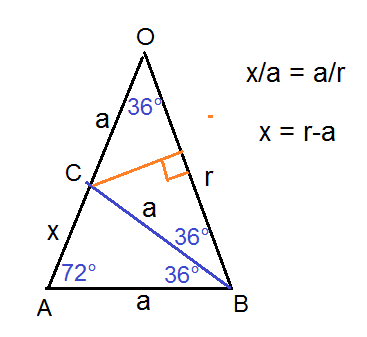# Trouble developing trig expression

• frkCarl
In summary, the conversation discusses an equation involving the cosine of 36 degrees and how to prove it without using the right side of the equation. The participants discuss the use of trigonometric manipulation and the golden ratio to solve the problem. Ultimately, it is shown that the equation is true for an angle of 36 degrees, which is also the exterior angle of a pentagon. f

## Homework Statement

It's not a problem per se, I'm just trying something, so there's no statement. What I'm trying to do it's to prove the forllowing equation but without using the member of the right.

(cos(36º) + 1)²/(cos(36º)) = 5cos(36º)

There's the trivial answer, using both members, that it's multiply both sides by cos(36º), call it x and solve the 2nd degree equation and get the positive result. I'm not trying to do that. I'm trying to develop (cos(36º) + 1)²/(cos(36º)) and turn it into 5cos(36º).

I'm not sure.

## The Attempt at a Solution

For simplicity sake, let 36º = u

(cos(u) + 1)²/(cos(u))
Developing and using sin^2(u) + cos^2(u) = 1 -> [cos^2(u) + 2cos(u) + 1]/cos(u)⇔ 2cos^2(u)+2cos(u)+sin^2(x)/cos(u)

Using the double angle for cosine, cos(2x) = cos^2(u) - sin^2(u):
3cos^2(u)-cos(2u)+2cos(u) / cos(u) ⇔ (3cos^2(u)/cos(u)) + (-cos(2u)+2cos(u))/cos(u)

Here's where I got stuck. I got everything in terms of cosine but the (-cos(2u)+2cos(u))/cos(u)) is giving me some trouble. How do I handle it?

Perhaps try plotting y = (cos(x) + 1)²/(cos(x)) - 5cos(x) and see if it crosses the x axis. If so, is it true for all x or just some values of x ?
Many software packages (including Excel) will treat the argument of cosine() as in radians. So keep that in mind.

If it is true for all x, then yes you can use Trig manipulation to make one expression into the other. If it is only true for some angles, then no you cannot.

•Mark44
These links are very helpful, I believe. The 36° angle is the exterior angle for a pentagon. In the second link, they show that ##\cos 36° = \frac {1 + \sqrt 5} 4##, which is probably the key piece in proving the identity.

Perhaps try plotting y = (cos(x) + 1)²/(cos(x)) - 5cos(x) and see if it crosses the x axis. If so, is it true for all x or just some values of x ?
Many software packages (including Excel) will treat the argument of cosine() as in radians. So keep that in mind.

If it is true for all x, then yes you can use Trig manipulation to make one expression into the other. If it is only true for some angles, then no you cannot.
The equation you wrote above isn't an identity, but it is true for an angle of 36° (or ##\frac \pi 5##).

Written a regular decadon into a circle of radius r, it is easy to prove that the side of the decagon is ##a = \frac{1}{2}\left(-1+\sqrt{5}\right)##
Consider the isoceles triangle ABO. The angle at the vertex is 36° and the angles at the base are of 72°. Halve one of the 72°angle, draw the ray, it crosses the side AO at point C. ABO triangle is similar to ABC triangle, so ##\frac {r-a}{a}=\frac{a}{r}##, which results in ##a = \frac{1}{2}\left(-1+\sqrt{5}\right)##
##\cos 36°= \frac{r}{2a} =\frac {1+\sqrt 5 }{4}###### Attachments

•